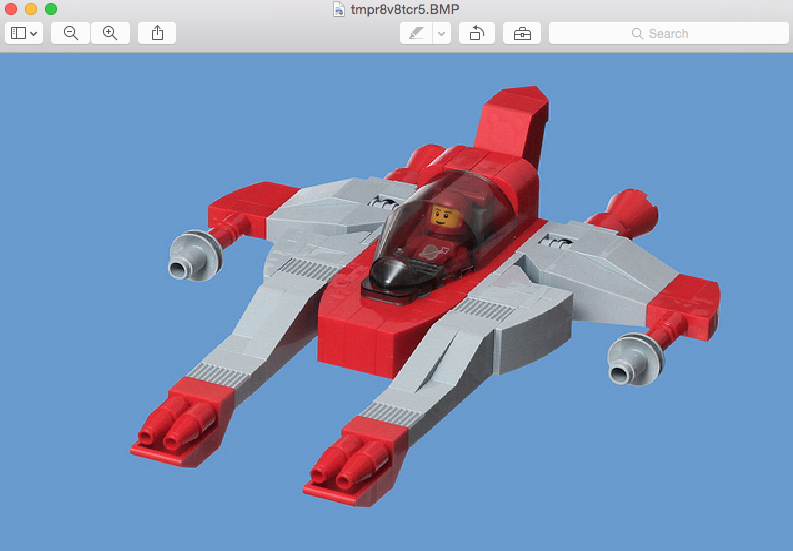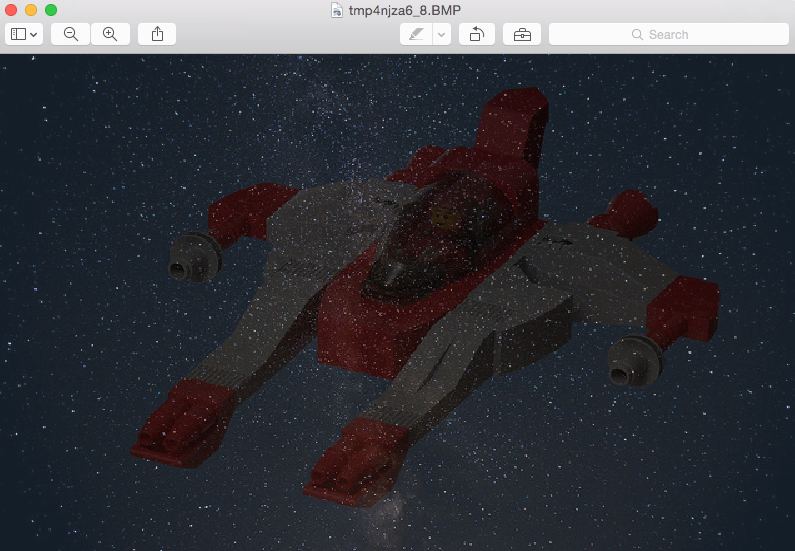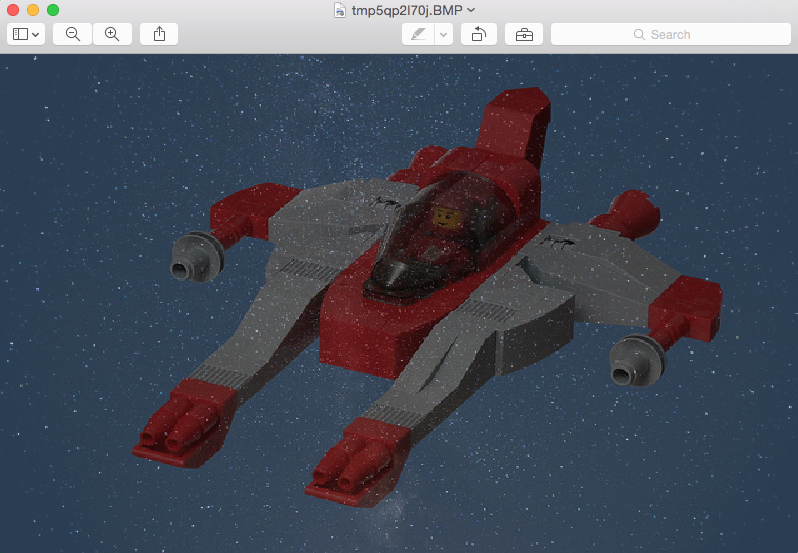# Blend Two Images Using An Alpha Value

## Overview:

• An alpha channel in an image introduces transparency to the Image.

• Alpha blending combines two images by applying an alpha value to the images.

• Pillow –Python Image Processing Library provides blend() method as part of the Image class implementation.

• When Image1 and Image2 are blended using alpha value 0, Image1 is returned as and vice versa when the alpha value is 1.

• When the alpha value varies from 0 to 1 and beyond, interpolation is used to determine the color value given by the formula

out=((int) in1[x] + alpha * ((int) in2[x] - (int) in1[x]));

## Example:

 from PIL import Image # Function to change the image size def changeImageSize(maxWidth,                      maxHeight,                      image):          widthRatio  = maxWidth/image.size     heightRatio = maxHeight/image.size     newWidth    = int(widthRatio*image.size)     newHeight   = int(heightRatio*image.size)     newImage    = image.resize((newWidth, newHeight))     return newImage      # Take two images for blending them together    image1 = Image.open("./sky1.png") image2 = Image.open("./spaceship1.png") # Make the images of uniform size image3 = changeImageSize(800, 500, image1) image4 = changeImageSize(800, 500, image2) # Make sure images got an alpha channel image5 = image3.convert("RGBA") image6 = image4.convert("RGBA") # Display the images image5.show() image6.show() # alpha-blend the images with varying values of alpha alphaBlended1 = Image.blend(image5, image6, alpha=.2) alphaBlended2 = Image.blend(image5, image6, alpha=.4) # Display the alpha-blended images alphaBlended1.show() alphaBlended2.show()

## Output:

### Image2 used in blending:### Alpha blended Image - alpha=0.2:### Alpha blended Image - alpha=0.4: